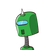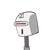# The cost of two pizzas is Rs. 198. Find the cost of 15 pizzas. ​

The cost of two pizzas is Rs. 198. Find the cost of 15 pizzas. ​

### 2 thoughts on “The cost of two pizzas is Rs. 198. Find the cost of 15 pizzas. ​”

1.Rs1485

Step-by-step explanation:

2 pizzas=Rs198

15pizzas

15×198÷2=1485

=Rs1485

2.